# US Supreme Court

This article provides an example of using `geom_braid()` with a flipped set of aesthetics `y`, `xmin`, `xmax` instead of the usual `x`, `ymin`, `ymax`. This change is primarily motivated by the fact that we are visualizing judicial ideology scores, from strongly liberal to strongly conservative, and we are accustomed to interpreting ideologies on the left-right political spectrum.

How has the ideology of the US Supreme Court changed over time?

A common way to measure judicial ideology is to use Martin-Quinn scores.

Developed by political science researchers Andrew Martin and Kevin Quinn in 2002, a Martin-Quinn score is a summary of a justice’s voting record in a given year using a Bayesian model. Positive scores indicate a conservative ideology, negative scores indicate a liberal ideology. The further a score is from zero, the stronger the ideology.

In this article, we’ll work with a couple datasets that Martin and Quinn maintain at mqscores.lsa.umich.edu.

## Court Ideology

We’ll start with `court.csv`, which provides the Martin-Quinn score of a hypothetical “median justice” each year since 1937.

``````library(ggplot2)
library(ggbraid)
library(dplyr)

"https://mqscores.lsa.umich.edu/media/2020/court.csv",
col_types = cols_only(term = col_character(), med = col_double())
) %>%
mutate(year = as.integer(substr(term, 1, 4))) %>%
group_by(year) %>%
summarize(mqscore = median(med), .groups = "drop") %>%
arrange(desc(year))

court
#> # A tibble: 84 × 2
#>     year mqscore
#>    <int>   <dbl>
#>  1  2020   0.607
#>  2  2019   0.303
#>  3  2018   0.371
#>  4  2017   0.293
#>  5  2016  -0.058
#>  6  2015  -0.272
#>  7  2014  -0.224
#>  8  2013   0.066
#>  9  2012   0.244
#> 10  2011   0.302
#> # … with 74 more rows``````

Let’s plot the data in `court` by mapping `year` to `x`, `mqscore` to `y`, and applying the line geometry with `geom_line()`. Also, place a horizontal line at `yintercept = 0` with `geom_hline()` to better identify the point at which an ideology

``````ggplot(court, aes(x = year, y = mqscore)) +
geom_line() +
geom_hline(yintercept = 0)``````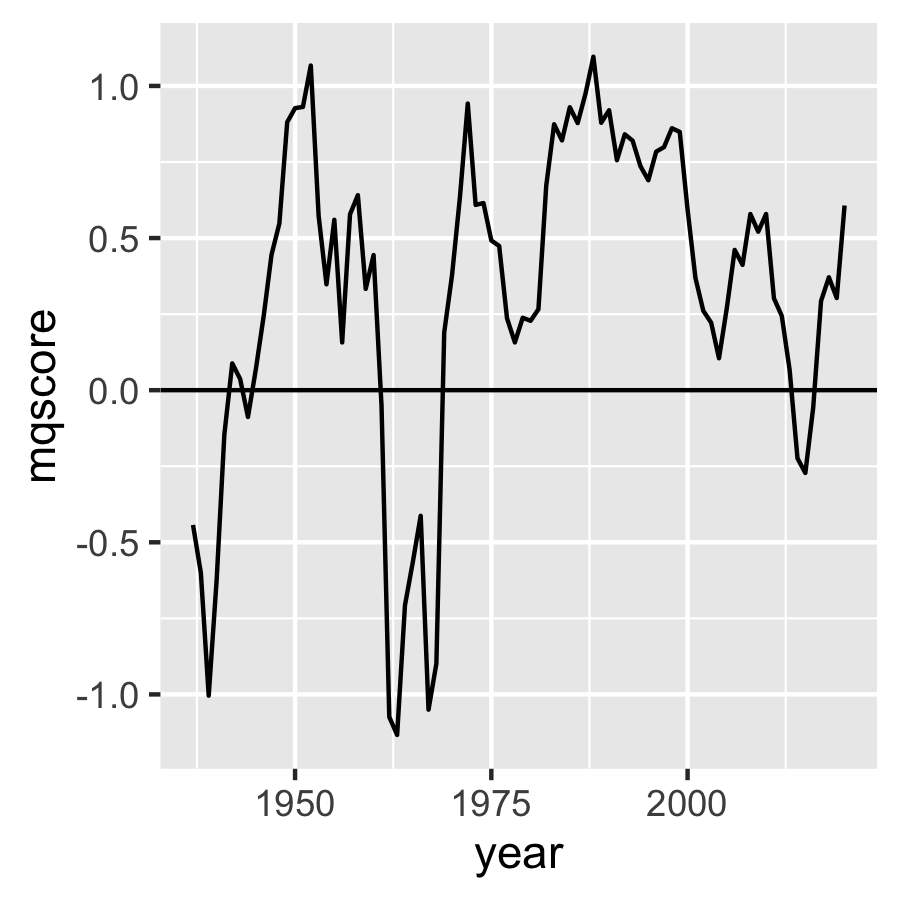A nice, simple plot.

Another way to convey this relationship is to instead map `mqscore` to `x` and `year` to `y`. With this mapping, Martin-Quinn scores that indicate a conservative ideology are to the right of zero and scores that indicate a liberal ideology are to the left. This matches the left-right political spectrum that we are accustomed to, although it does put a temporal variable on the y axis which can feel strange.

In any case, let’s give it a try.

Starting with the previous code, switch `year` with `mqscore`, and replace `geom_hline()` with `geom_vline()` and `yintercept = 0` with `xintercept = 0`.

``````ggplot(court, aes(x = mqscore, y = year)) +
geom_line() +
geom_vline(xintercept = 0)``````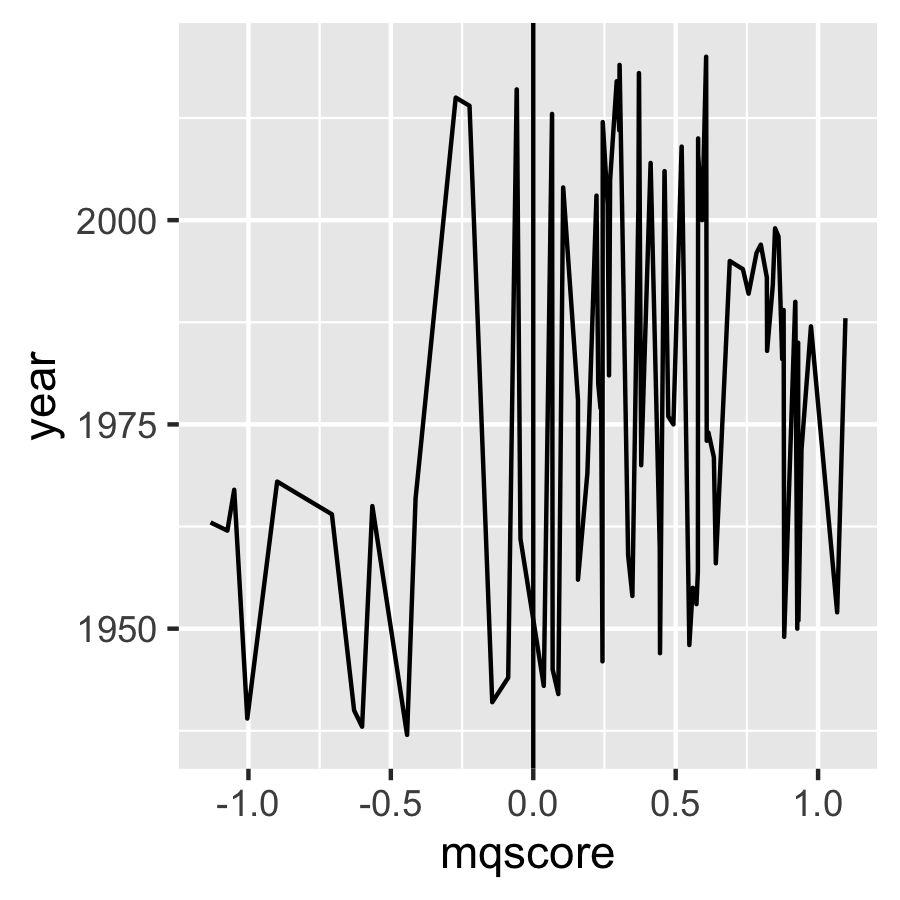Hmmm, that isn’t right. What’s gone wrong?

The problem is that `geom_line()` connects observations in the order of the variable on the x axis. So, because we have mapped `mqscore` to `x`, `geom_line()` orders the data by `mqscore` before drawing lines between observations. That’s not what we want, we’d rather the geom leave the order as is.

To avoid this ordering behavior, we can use `geom_path()` in place of `geom_line()`.

``````ggplot(court, aes(x = mqscore, y = year)) +
geom_path() +
geom_vline(xintercept = 0)``````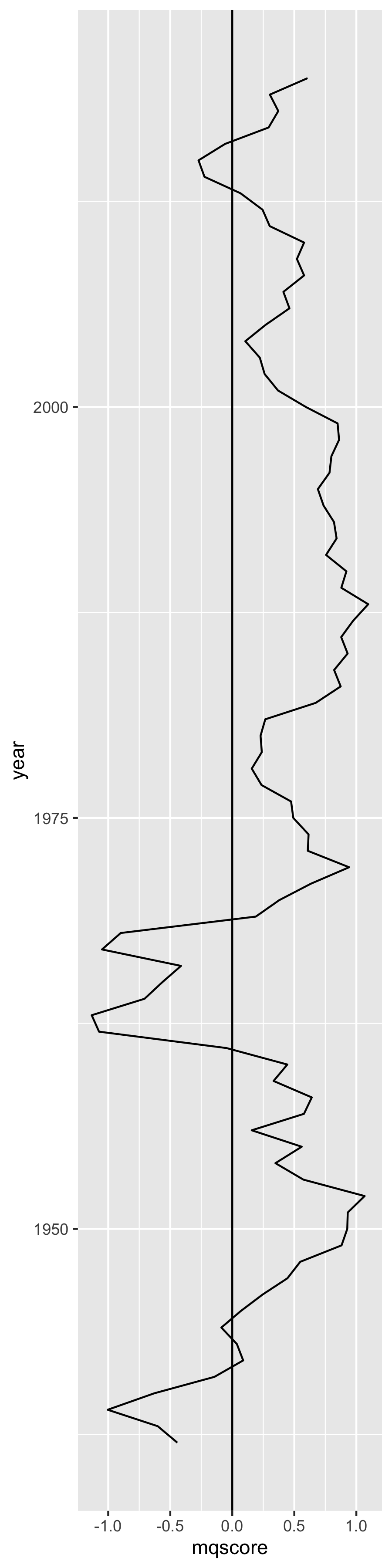Much better.

But this plot could really use some color. We can use `geom_braid()` to fill the area between the “median justice” Martin-Quinn score line and the x intercept at 0.

``````ggplot(court, aes(x = mqscore, y = year)) +
geom_path() +
geom_vline(xintercept = 0) +
geom_braid(
aes(xmin = mqscore, xmax = 0, fill = mqscore < 0),
method = "line"
)``````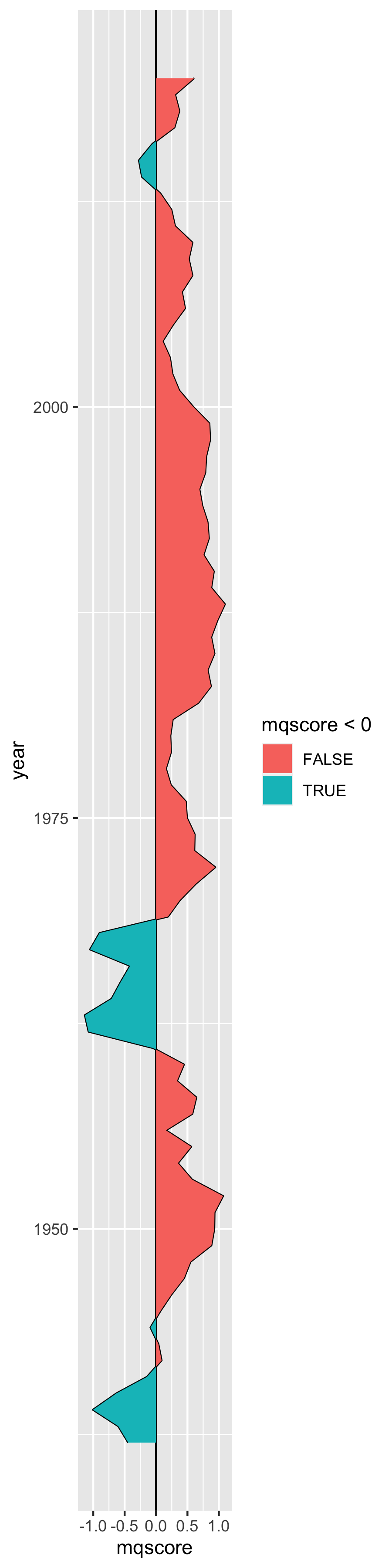Touching this up a bit, we can remove the vertical line at zero because it is no longer necessary, and we can remove the `fill` aesthetic legend because it doesn’t add any value. While we’re at it, let’s change the colors and add some transparency.

``````ggplot(court, aes(x = mqscore, y = year)) +
geom_path() +
geom_braid(
aes(xmin = mqscore, xmax = 0, fill = mqscore < 0),
method = "line",
alpha = 0.3
) +
scale_fill_manual(values = c("red", "blue")) +
guides(fill = "none")``````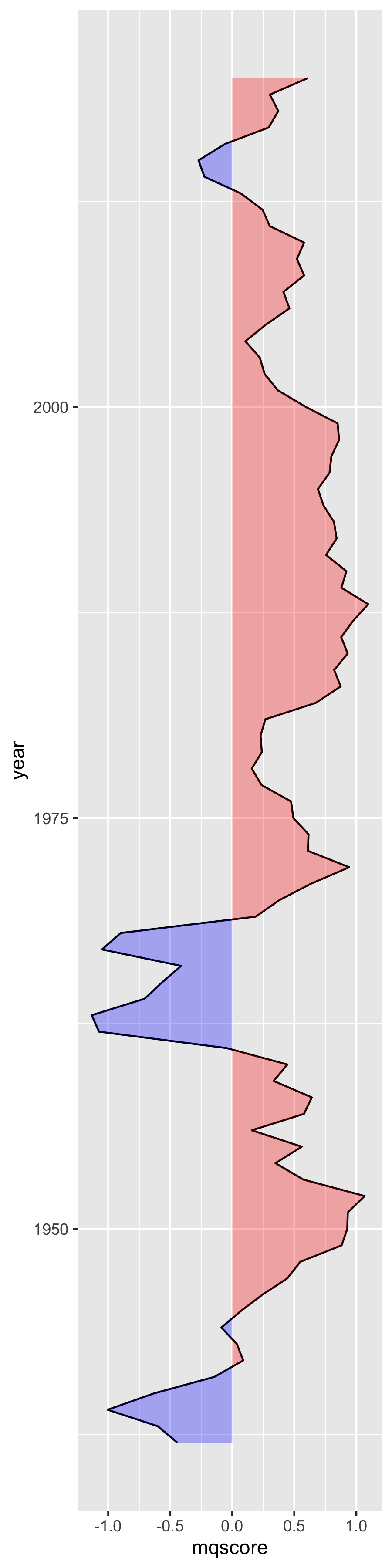Voila.

## Justice Ideology

Now it may also be valuable to include data from `justices.csv`, which provides the Martin-Quinn score of every justice each year since 1937.

``````justices <- read_csv(
"https://mqscores.lsa.umich.edu/media/2020/justices.csv",
col_types = cols_only(
term = col_integer(),
justiceName = col_character(),
post_med = col_double()
)
) %>%
rename(year = term, name = justiceName, mqscore = post_med) %>%
arrange(desc(year), name)

justices
#> # A tibble: 764 × 3
#>     year name        mqscore
#>    <int> <chr>         <dbl>
#>  1  2020 ACBarrett     0.981
#>  2  2020 BMKavanaugh   0.539
#>  3  2020 CThomas       3
#>  4  2020 EKagan       -1.49
#>  5  2020 JGRoberts     0.498
#>  6  2020 NMGorsuch     1.1
#>  7  2020 SAAlito       2.15
#>  8  2020 SGBreyer     -1.89
#>  9  2020 SSotomayor   -3.94
#> 10  2019 BMKavanaugh   0.552
#> # … with 754 more rows``````

Let’s add individual Martin-Quinn score lines for every justice.

``````ggplot(court, aes(x = mqscore, y = year)) +
geom_path() +
geom_braid(
aes(xmin = mqscore, xmax = 0, fill = mqscore < 0),
method = "line",
alpha = 0.3
) +
geom_path(aes(group = name), data = justices, size = 0.3, alpha = 0.2) +
scale_fill_manual(values = c("red", "blue")) +
guides(fill = "none")``````